Back to Contents

# Growing Trees

We have used lists to represent collections of elements of like type but varying length, and tuples to represent collections of things of any type but fixed length. Another common type is the binary tree, which is used to represent structures which branch, such as the arithmetical expressions we constructed in the last chapter.

How can we represent such trees using an OCaml type? When we built our version of the OCaml list type, we had two constructors – `Cons` to hold a head and a tail, and `Nil` to represent the end of the list. With a tree, we need a version of Cons which can hold two tails – the left and right, and we still need a version of `Nil`.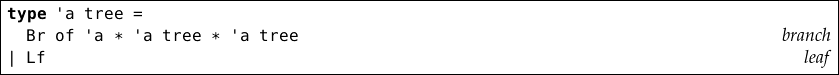Our type is called tree, and is polymorphic (can hold any kind of data at the branches). There are two constructors: `Br` for branches, which hold three things in a tuple: an element, the left sub-tree, and the right sub-tree. If it is not a `Br`, it is a `Lf` (leaf), which is used to signal that there is no left, or no right sub-tree. Here are some representations in our new type of integer trees: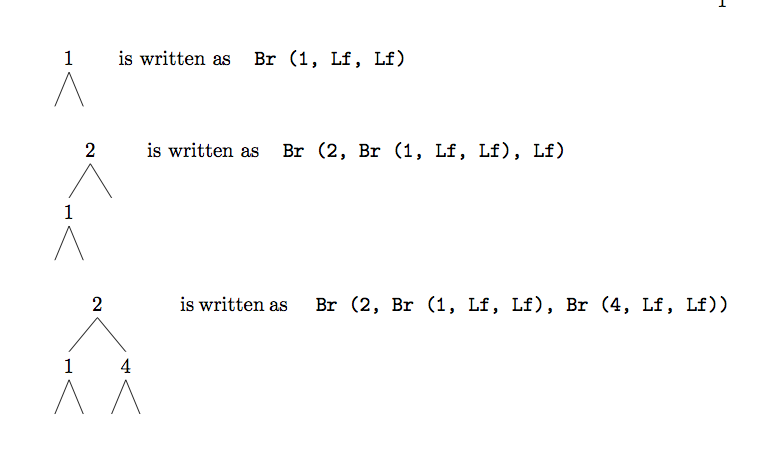The empty tree is simply `Lf`. You can see now why we used abbreviated constructor names – even small trees result in long textual representations. Let us write some simple functions on trees. To calculate the number of elements in the tree, we just count one for each branch, and zero for each leaf: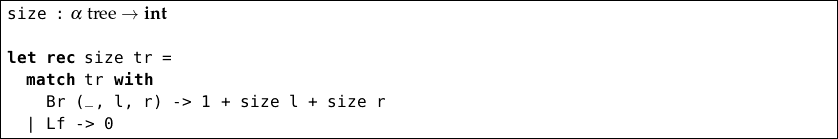Notice that the recursive function follows the shape of the recursive type. A similar function can be used to add up all the integers in an int tree: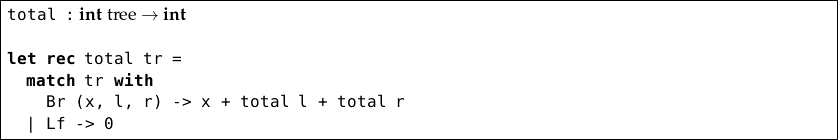How can we calculate the maximum depth of a tree? The depth is the longest path from the root (top) of the tree to a leaf.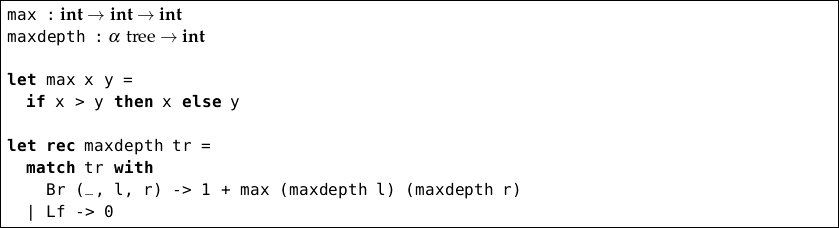We defined a function `max` which returns the larger of two integers. Then, in our main function, we count a leaf as zero depth, and calculate the depth of a branch as one plus the maximum of the left and right sub-trees coming from that branch. Now consider extracting all of the elements from a tree into a list: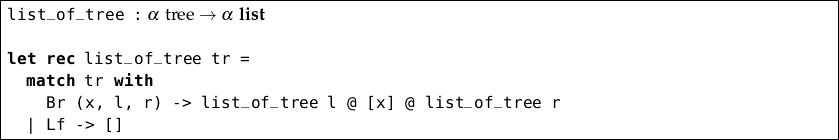Notice that we chose to put all the elements on the left branch before the current element, and all the elements in the right branch after. This is arbitrary (it is clear that there are multiple answers to the question “How can I extract all the elements from a tree as a list?”). Before we consider real applications of trees, let us look at one more function. Here is how to map over trees: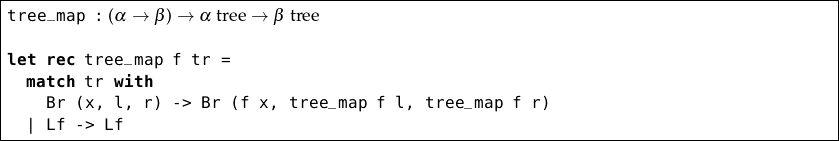Notice the similarity to our `map` function for lists, both in the type and the definition.

## Using trees to build better dictionaries

We have seen that arithmetic expressions can be drawn as trees on paper, and we have designed an OCaml data type for binary trees to hold any kind of element. Now it is time to introduce the most important application of trees: the binary search tree, which is another way of implementing the dictionary data structure we described in Chapter 7.

The most important advantage of a tree is that it is often very much easier to reach a given element. When searching in a dictionary defined as a list, it took on average time proportional to the number of items in the dictionary to find a value for a key (the position of the required entry is, on average, halfway along the list). If we use a binary tree, and if it is reasonably nicely balanced in shape, that time can be reduced to the logarithm base two of the number of elements in the dictionary. Can you see why?

We can use our existing tree type. In the case of a dictionary, it will have type (α × β) tree, in other words a tree of key-value pairs where the keys have some type α and the values some type β. For this example, we are going to be using another built-in type, string. A string is a sequence of characters written between double quotation marks. We have seen these as messages attached to exceptions, but they are a basic OCaml type too.

So, our tree representing a dictionary mapping integers like `1` to their spellings like `“one”` would have type (int × string) tree: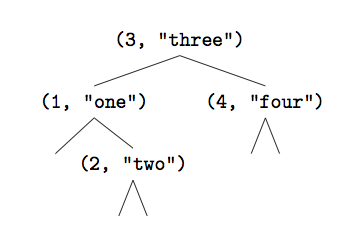which would be written as

`Br ((3, "three"), Br ((1, "one"), Lf, Br ((2, "two"), Lf, Lf)), Br ((4, "four"), Lf, Lf))`

If we arrange the tree such that, at each branch, everything to the left has a key less than the key at the branch, and everything to the right has a key greater than that at the branch, we have a binary search tree.

Lookup is simple: start at the top, and if we have not found the key we are looking for, go left or right depending upon whether the required key is smaller or larger than the value at the current branch. If we reach a leaf, the key was not in the tree (assuming the tree is a well-formed binary search tree), and we raise an exception.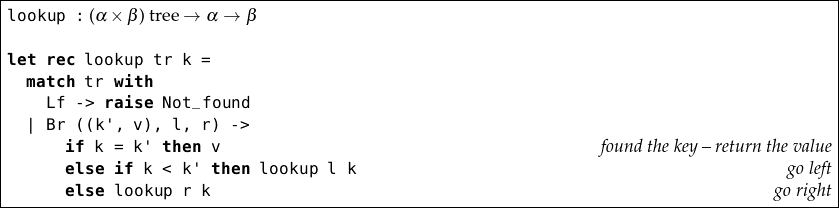Alternatively, we may use the option type to avoid exceptions: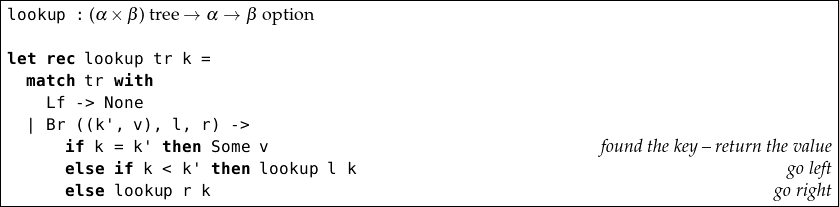How can we insert a new key-value pair into an existing tree? We can find the position to insert by using the same procedure as the lookup function – going left or right at each branch as appropriate. If we find an equal key, we put our new value there instead. Otherwise, we will end up at a leaf, and this is the insertion point – thus, if the key is not in the dictionary when `insert` is used, it will be added in place of an existing leaf.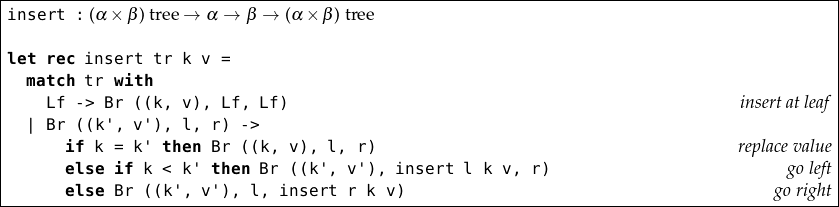For example, if we wish to insert the value `"zero"` for the key `0` in the tree drawn above, we would obtain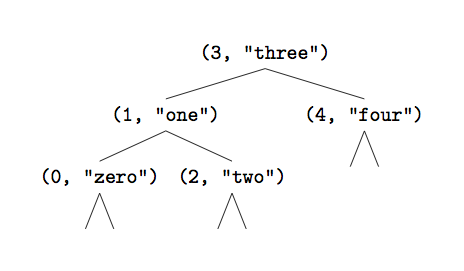The shape of the tree is dependent upon the order of insertions into the tree – if they are in order (or reverse order), we obtain a rather inefficient tree – no better a dictionary than a list in fact. However, on average, we obtain a reasonably balanced tree, and logarithmic lookup and insertion times.

Lists and trees are examples of data structures. The design of an algorithm and its data structures are intimately connected.

## Questions

1. Write a function of type α α tree bool to determine if a given element is in a tree.

2. Write a function which flips a tree left to right such that, if it were drawn on paper, it would appear to be a mirror image.

3. Write a function to determine if two trees have the same shape, irrespective of the actual values of the elements.

4. Write a function `tree_of_list` which builds a tree representation of a dictionary from a list representation of a dictionary.

5. Write a function to combine two dictionaries represented as trees into one. In the case of clashing keys, prefer the value from the first dictionary.

6. Can you define a type for trees which, instead of branching exactly two ways each time, can branch zero or more ways, possibly different at each branch? Write simple functions like `size`, `total`, and `map` using your new type of tree.